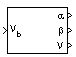# Incidence, Sideslip, & Airspeed

Calculate incidence, sideslip, and airspeed

• Library:
• Aerospace Blockset / Flight Parameters

•## Description

The Incidence, Sideslip, & Airspeed block calculates the angles between the velocity vector and the body, and also the total airspeed from the velocity components in the body-fixed coordinate frame. For the equations used in the calculation, see Algorithms.

## Ports

### Input

expand all

Velocity of the body, specified as a three-element vector, resolved into the body-fixed coordinate frame.

Data Types: `double`

### Output

expand all

Incidence angle, returned as a scalar, in radians.

Data Types: `double`

Sideslip angle, returned as a scalar, in radians.

Data Types: `double`

Airspeed of the body, returned as a scalar.

Data Types: `double`

## Algorithms

To calculate the angles between the velocity vector and the body, and the total airspeed, the block uses these equations:

`$\alpha =a\mathrm{tan}\left(\frac{w}{u}\right)$`
`$\beta =a\mathrm{sin}\left(\frac{v}{V}\right)$`
`$V=\sqrt{{u}^{2}+{v}^{2}+{w}^{2}}$`
``

## Extended Capabilities

### C/C++ Code GenerationGenerate C and C++ code using Simulink® Coder™.